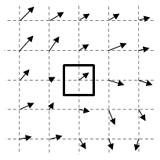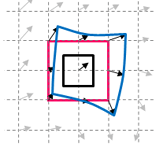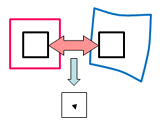# Algorithms of KoncertoⅡ-1

## KoncertoⅡ supports newest PIV analysis algorithms

Seika Digital Image has closely collaborated with world leading research institutes, and has continuously added support for the newest technology to the KoncertoⅡ.

### Standard FFT cross correlation

Basic displacement (velocity) evaluation method. Almost the same results as direct cross correlation are obtained in much shorter calculation time.

### Multi pass cross correlation

Apply standard cross correlation at first step. Then shift the interrogation window of second image based on the predictor (displacement vector obtained by the previous process of standard cross correlation). And then apply cross correlation again. The smaller displacement is obtained this time. After applying this operation several times iteratively, the resulting displacement becomes nearly zero. The final displacement is the sum of all displacements in previous processes.

### Multi grid cross correlation

Multi grid cross correlation decreases the interrogation window size with each iteration of the multi pass operation. The advantage of the multi grid scheme is to obtain the higher spatial resolution and/or wider velocity dynamic range.

### Central difference Interrogation

In the iterative cross correlation processing, the cross correlation between the interrogation windows of the 1st image is moved 1/2 backward of the predictor value (displacement obtained by the standard cross correlation) and the interrogation windows of 2nd image is moved 1/2 forward of the predictor. This operation results in the second order accurate in PIV analysis.

### Double (multi) correlation

Multiplying the correlation planes of 2 adjacent interrogation windows increases the orderly signal and reduces the random noise, resulting in the increase of signal to noise ratio.

### Image deformation correlation

In the iterative processing, images are deformed based on the surrounding predictors by spline function. Applying this operation iteratively several times will deform the resulting second image almost identical to the first image.
The resulting displacement becomes nearly zero. And then more accurate results are obtained. This technique is effective even if there is a rotation or deformation in the interrogation window. This is also effective for reducing so-called "peak locking error".

### Average correlation

This operation is effective with noisy images such as micro PIV. It also reduces the noise from the Brownian motion. This technique is only applicable in average analysis, not in instantaneous analysis.

### Concept of Image deformation correlation

Determine the value of surrounding predictor vectors by cross correlation.
Deform the shape of interrogation window on the second image (blue) based on surrounding predictors.Determine the value of surrounding predictor vectors by cross correlation. Deform the shape of interrogation window on the second image (blue) based on surrounding predictors. Apply cross correlation again between black windows. The smaller displacement than the previous process is obtained.